xMolecular HamiltonianEncyclopedia
In atomic, molecular, and optical physics
Atomic, molecular, and optical physics
Atomic, molecular, and optical physics is the study of matter-matter and light-matter interactions on the scale of single atoms or structures containing a few atoms. The three areas are grouped together because of their interrelationships, the similarity of methods used, and the commonality of the...

as well as in quantum chemistry
Quantum chemistry
Quantum chemistry is a branch of chemistry whose primary focus is the application of quantum mechanics in physical models and experiments of chemical systems...

, molecular Hamiltonian is the name given to the Hamiltonian
Hamiltonian (quantum mechanics)
In quantum mechanics, the Hamiltonian H, also Ȟ or Ĥ, is the operator corresponding to the total energy of the system. Its spectrum is the set of possible outcomes when one measures the total energy of a system...

representing the energy
Energy
In physics, energy is an indirectly observed quantity. It is often understood as the ability a physical system has to do work on other physical systems...

of the electron
Electron
The electron is a subatomic particle with a negative elementary electric charge. It has no known components or substructure; in other words, it is generally thought to be an elementary particle. An electron has a mass that is approximately 1/1836 that of the proton...

s and nuclei
Atomic nucleus
The nucleus is the very dense region consisting of protons and neutrons at the center of an atom. It was discovered in 1911, as a result of Ernest Rutherford's interpretation of the famous 1909 Rutherford experiment performed by Hans Geiger and Ernest Marsden, under the direction of Rutherford. The...

in a molecule
Molecule
A molecule is an electrically neutral group of at least two atoms held together by covalent chemical bonds. Molecules are distinguished from ions by their electrical charge...

. This Hermitian operator
In mathematics, on a finite-dimensional inner product space, a self-adjoint operator is an operator that is its own adjoint, or, equivalently, one whose matrix is Hermitian, where a Hermitian matrix is one which is equal to its own conjugate transpose...

and the associated Schrödinger equation
Schrödinger equation
The Schrödinger equation was formulated in 1926 by Austrian physicist Erwin Schrödinger. Used in physics , it is an equation that describes how the quantum state of a physical system changes in time....

play a central role in computational chemistry
Computational chemistry
Computational chemistry is a branch of chemistry that uses principles of computer science to assist in solving chemical problems. It uses the results of theoretical chemistry, incorporated into efficient computer programs, to calculate the structures and properties of molecules and solids...

and physics
Computational physics
Computational physics is the study and implementation of numerical algorithms to solve problems in physics for which a quantitative theory already exists...

for computing properties of molecules and aggregates of molecules, such as thermal conductivity
Thermal conductivity
In physics, thermal conductivity, k, is the property of a material's ability to conduct heat. It appears primarily in Fourier's Law for heat conduction....

, specific heat, electrical conductivity, optical
Optics
Optics is the branch of physics which involves the behavior and properties of light, including its interactions with matter and the construction of instruments that use or detect it. Optics usually describes the behavior of visible, ultraviolet, and infrared light...

, and magnetic properties
Magnetism
Magnetism is a property of materials that respond at an atomic or subatomic level to an applied magnetic field. Ferromagnetism is the strongest and most familiar type of magnetism. It is responsible for the behavior of permanent magnets, which produce their own persistent magnetic fields, as well...

, and reactivity.

The elementary parts of a molecule are the nuclei, characterized by their atomic numbers, Z, and the electrons, which have negative elementary charge
Elementary charge
The elementary charge, usually denoted as e, is the electric charge carried by a single proton, or equivalently, the absolute value of the electric charge carried by a single electron. This elementary charge is a fundamental physical constant. To avoid confusion over its sign, e is sometimes called...

, -e. Their interaction gives a nuclear charge of Z+q, where q=-eN, with N equal to the number of electrons. Electrons and nuclei are, to a very good approximation, point charges and point masses. The molecular Hamiltonian is a sum of several terms: its major terms are the kinetic energies
Kinetic energy
The kinetic energy of an object is the energy which it possesses due to its motion.It is defined as the work needed to accelerate a body of a given mass from rest to its stated velocity. Having gained this energy during its acceleration, the body maintains this kinetic energy unless its speed changes...

of the electrons and the Coulomb (electrostatic) interactions
Coulomb's law
Coulomb's law or Coulomb's inverse-square law, is a law of physics describing the electrostatic interaction between electrically charged particles. It was first published in 1785 by French physicist Charles Augustin de Coulomb and was essential to the development of the theory of electromagnetism...

between the two kinds of charged particles. The Hamiltonian that contains only the kinetic energies of electrons and nuclei, and the Coulomb interactions between them, is known as the Coulomb Hamiltonian. From it are missing a number of small terms, most of which are due to electronic and nuclear spin
Spin (physics)
In quantum mechanics and particle physics, spin is a fundamental characteristic property of elementary particles, composite particles , and atomic nuclei.It is worth noting that the intrinsic property of subatomic particles called spin and discussed in this article, is related in some small ways,...

.

Although it is generally assumed that the solution of the time-independent Schrödinger equation
Schrödinger equation
The Schrödinger equation was formulated in 1926 by Austrian physicist Erwin Schrödinger. Used in physics , it is an equation that describes how the quantum state of a physical system changes in time....

associated with the Coulomb Hamiltonian will predict most properties of the molecule, including its shape (three-dimensional structure), calculations based on the full Coulomb Hamiltonian are very rare. The main reason is that its Schrödinger equation is very difficult to solve. Applications are restricted to small systems like the hydrogen molecule.

Almost all calculations of molecular wavefunctions are based on the separation of the Coulomb Hamiltonian first devised by Born and Oppenheimer
Born-Oppenheimer approximation
In quantum chemistry, the computation of the energy and wavefunction of an average-size molecule is a formidable task that is alleviated by the Born–Oppenheimer approximation, named after Max Born and J. Robert Oppenheimer. For instance the benzene molecule consists of 12 nuclei and 42...

. The nuclear kinetic energy terms are omitted from the Coulomb Hamiltonian and one considers the remaining Hamiltonian as a Hamiltonian of electrons only. The stationary nuclei enter the problem only as generators of an electric potential in which the electrons move in a quantum mechanical way. Within this framework the molecular Hamiltonian has been simplified to the so-called clamped nucleus Hamiltonian, also called electronic Hamiltonian, that acts only on functions of the electronic coordinates.

Once the Schrödinger equation of the clamped nucleus Hamiltonian has been solved for a sufficient number of constellations of the nuclei, an appropriate eigenvalue (usually the lowest) can be seen as a function
Function (mathematics)
In mathematics, a function associates one quantity, the argument of the function, also known as the input, with another quantity, the value of the function, also known as the output. A function assigns exactly one output to each input. The argument and the value may be real numbers, but they can...

of the nuclear coordinates, which leads to a potential energy surface
Potential energy surface
A potential energy surface is generally used within the adiabatic or Born–Oppenheimer approximation in quantum mechanics and statistical mechanics to model chemical reactions and interactions in simple chemical and physical systems...

. In practical calculations the surface is usually fitted
Least squares
The method of least squares is a standard approach to the approximate solution of overdetermined systems, i.e., sets of equations in which there are more equations than unknowns. "Least squares" means that the overall solution minimizes the sum of the squares of the errors made in solving every...

in terms of some analytic functions. In the second step of the Born-Oppenheimer approximation
Born-Oppenheimer approximation
In quantum chemistry, the computation of the energy and wavefunction of an average-size molecule is a formidable task that is alleviated by the Born–Oppenheimer approximation, named after Max Born and J. Robert Oppenheimer. For instance the benzene molecule consists of 12 nuclei and 42...

the part of the full Coulomb Hamiltonian that depends on the electrons is replaced by the potential energy surface. This converts the total molecular Hamiltonian into another Hamiltonian that acts only on the nuclear coordinates. In the case of a breakdown of the Born-Oppenheimer approximation
Born-Oppenheimer approximation
In quantum chemistry, the computation of the energy and wavefunction of an average-size molecule is a formidable task that is alleviated by the Born–Oppenheimer approximation, named after Max Born and J. Robert Oppenheimer. For instance the benzene molecule consists of 12 nuclei and 42...

—which occurs when energies of different electronic states are close—the neighboring potential energy surfaces are needed, see this article
Born-Oppenheimer approximation
In quantum chemistry, the computation of the energy and wavefunction of an average-size molecule is a formidable task that is alleviated by the Born–Oppenheimer approximation, named after Max Born and J. Robert Oppenheimer. For instance the benzene molecule consists of 12 nuclei and 42...

for more details on this.

The nuclear motion Schrödinger equation can be solved in a space-fixed (laboratory) frame
Frame of reference
A frame of reference in physics, may refer to a coordinate system or set of axes within which to measure the position, orientation, and other properties of objects in it, or it may refer to an observational reference frame tied to the state of motion of an observer.It may also refer to both an...

, but then the translation
Translation (physics)
In physics, translation is movement that changes the position of an object, as opposed to rotation. For example, according to Whittaker:...

al and rotation
Rotation
A rotation is a circular movement of an object around a center of rotation. A three-dimensional object rotates always around an imaginary line called a rotation axis. If the axis is within the body, and passes through its center of mass the body is said to rotate upon itself, or spin. A rotation...

al (external) energies are not accounted for. Only the (internal) atomic vibration
Vibration
Vibration refers to mechanical oscillations about an equilibrium point. The oscillations may be periodic such as the motion of a pendulum or random such as the movement of a tire on a gravel road.Vibration is occasionally "desirable"...

s enter the problem. Further, for molecules larger than triatomic ones, it is quite common to introduce the harmonic approximation, which approximates the potential energy surface as a quadratic function
A quadratic function, in mathematics, is a polynomial function of the formf=ax^2+bx+c,\quad a \ne 0.The graph of a quadratic function is a parabola whose axis of symmetry is parallel to the y-axis....

of the atomic displacements. This gives the harmonic nuclear motion Hamiltonian. Making the harmonic approximation, we can convert the Hamiltonian into a sum of uncoupled one-dimensional harmonic oscillator
Harmonic oscillator
In classical mechanics, a harmonic oscillator is a system that, when displaced from its equilibrium position, experiences a restoring force, F, proportional to the displacement, x: \vec F = -k \vec x \, where k is a positive constant....

Hamiltonians. The one-dimensional harmonic oscillator is one of the few systems that allows an exact solution of the Schrödinger equation.

Alternatively, the nuclear motion (rovibrational) Schrödinger equation can be solved in a special frame (an Eckart frame
Eckart conditions
The Eckart conditions, named after Carl Eckart, sometimes referred to as Sayvetz conditions, simplify the nuclear motion Schrödinger equation that arises in the second step of the Born-Oppenheimer approximation. The Eckart conditions allow to a large extent the separation of the external ...

) that rotates and translates with the molecule. Formulated with respect to this body-fixed frame the Hamiltonian accounts for rotation
Rotation
A rotation is a circular movement of an object around a center of rotation. A three-dimensional object rotates always around an imaginary line called a rotation axis. If the axis is within the body, and passes through its center of mass the body is said to rotate upon itself, or spin. A rotation...

, translation
Translation
Translation is the communication of the meaning of a source-language text by means of an equivalent target-language text. Whereas interpreting undoubtedly antedates writing, translation began only after the appearance of written literature; there exist partial translations of the Sumerian Epic of...

and vibration
Vibration
Vibration refers to mechanical oscillations about an equilibrium point. The oscillations may be periodic such as the motion of a pendulum or random such as the movement of a tire on a gravel road.Vibration is occasionally "desirable"...

of the nuclei. Since Watson introduced in 1968 an important simplification to this Hamiltonian, it is often referred to as Watson's nuclear motion Hamiltonian, but it is also known as the Eckart Hamiltonian.

## Coulomb Hamiltonian

The algebraic form of many observables—i.e., Hermitian operators representing observable quantities—is obtained by the following quantization rules:
• Write the classical form of the observable in Hamilton form (as a function of momenta p and positions q). Both vectors are expressed with respect to an arbitrary inertial frame, usually referred to as laboratory-frame or space-fixed frame.
• Replace p by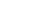and interpret q as a multiplicative operator. Here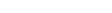is the nabla
Del
In vector calculus, del is a vector differential operator, usually represented by the nabla symbol \nabla . When applied to a function defined on a one-dimensional domain, it denotes its standard derivative as defined in calculus...

operator, a vector operator consisting of first derivatives. The well-known commutation relations for the p and q operators follow directly from the differentiation rules.

Classically the electrons and nuclei in a molecule have kinetic energy of the form p2/(2m) and
interact via Coulomb interactions
Coulomb's law
Coulomb's law or Coulomb's inverse-square law, is a law of physics describing the electrostatic interaction between electrically charged particles. It was first published in 1785 by French physicist Charles Augustin de Coulomb and was essential to the development of the theory of electromagnetism...

, which are inversely proportional to the distance rij
between particle i and j.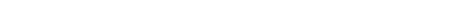In this expression ri stands for the coordinate vector of any particle (electron or nucleus). But from here on we will reserve capital R to represent the nuclear coordinate, and lower case r for the electrons of the system. The coordinates can be taken to be expressed with respect to any Cartesian frame centered anywhere in space, because distance, being an inner product, is invariant under rotation of the frame and, being the norm of a difference vector, distance is invariant under translation of the frame as well.

By quantizing the classical energy in Hamilton form one obtains the
a molecular Hamilton operator that is often referred to as the Coulomb Hamiltonian.
This Hamiltonian is a sum of 5 terms. They are
1. The kinetic energy operators for each nucleus in the system;
2. The kinetic energy operators for each electron in the system;
3. The potential energy between the electrons and nuclei - the total electron-nucleus Coulombic attraction in the system;
4. The potential energy arising from Coulombic electron-electron repulsions
5. The potential energy arising from Coulombic nuclei-nuclei repulsions - also known as the nuclear repulsion energy. See electric potential
Electric potential
In classical electromagnetism, the electric potential at a point within a defined space is equal to the electric potential energy at that location divided by the charge there...

for more details.

1.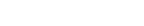2.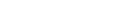3.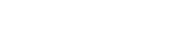4.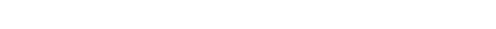1.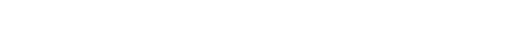Here Mi is the mass of nucleus i, Zi is the atomic number
Atomic number
In chemistry and physics, the atomic number is the number of protons found in the nucleus of an atom and therefore identical to the charge number of the nucleus. It is conventionally represented by the symbol Z. The atomic number uniquely identifies a chemical element...

of nucleus i, and me is the mass of the electron. The Laplace operator
Laplace operator
In mathematics the Laplace operator or Laplacian is a differential operator given by the divergence of the gradient of a function on Euclidean space. It is usually denoted by the symbols ∇·∇, ∇2 or Δ...

of particle i is :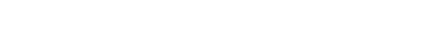. Since the kinetic energy operator is an inner product, it is invariant under rotation of the Cartesian frame with respect to which xi, yi, and zi are expressed. The kinetic energy operator, however, is not invariant under translation (choice of origin of the frame).

## Small terms

In the 1920s much spectroscopic evidence made it clear that the Coulomb Hamiltonian
is missing certain terms. Especially for molecules containing heavier atoms, these terms, although much smaller than kinetic and Coulomb energies, are nonnegligible. These spectroscopic observations led to the introduction of a new degree of freedom for electrons and nuclei, namely spin
Spin (physics)
In quantum mechanics and particle physics, spin is a fundamental characteristic property of elementary particles, composite particles , and atomic nuclei.It is worth noting that the intrinsic property of subatomic particles called spin and discussed in this article, is related in some small ways,...

. This empirical concept was given a theoretical basis by Paul Dirac
Paul Dirac
Paul Adrien Maurice Dirac, OM, FRS was an English theoretical physicist who made fundamental contributions to the early development of both quantum mechanics and quantum electrodynamics...

when he introduced a relativistically correct (Lorentz covariant) form of the one-particle Schrödinger equation. The Dirac equation predicts that spin and spatial motion of a particle interact via spin-orbit coupling
Spin-orbit interaction
In quantum physics, the spin-orbit interaction is any interaction of a particle's spin with its motion. The first and best known example of this is that spin-orbit interaction causes shifts in an electron's atomic energy levels due to electromagnetic interaction between the electron's spin and...

. In analogy spin-other-orbit coupling was introduced. The fact that particle spin has some of the characteristics of a magnetic dipole led to spin-spin coupling
Magnetic dipole-dipole interaction
Magnetic dipole–dipole interaction, also called dipolar coupling, refers to the direct interaction between two magnetic dipoles. The potential energy of the interaction is as follows:...

. Further terms without a classical counterpart are the Fermi-contact term (interaction of electronic
density on a finite size nucleus with the nucleus), and nuclear quadrupole coupling (interaction of a nuclear quadrupole
A quadrupole or quadrapole is one of a sequence of configurations of—for example—electric charge or current, or gravitational mass that can exist in ideal form, but it is usually just part of a multipole expansion of a more complex structure reflecting various orders of complexity.-Mathematical...

with the gradient of an electric field due to the electrons). Finally a parity violating term predicted by the Standard Model
Standard Model
The Standard Model of particle physics is a theory concerning the electromagnetic, weak, and strong nuclear interactions, which mediate the dynamics of the known subatomic particles. Developed throughout the mid to late 20th century, the current formulation was finalized in the mid 1970s upon...

must be mentioned. Although it is an extremely small interaction, it has attracted a fair amount of attention in the scientific literature because it gives different energies for the enantiomers in chiral molecules.

The remaining part of this article will ignore spin terms and consider the solution of the eigenvalue (time-independent Schrödinger) equation of the Coulomb Hamiltonian.

## The Schrödinger equation of the Coulomb Hamiltonian

The Coulomb Hamiltonian has a continuous spectrum due to the center of mass motion of the molecule in homogeneous space. In classical mechanics it is easy to separate off the center of mass
Center of mass
In physics, the center of mass or barycenter of a system is the average location of all of its mass. In the case of a rigid body, the position of the center of mass is fixed in relation to the body...

(COM) motion of a system of point masses. Classically the motion of the COM is uncoupled from the other motions. The COM moves uniformly (i.e., with constant velocity) through space as if it were a point particle with mass equal to the sum Mtot of the masses of all the particles.

In quantum mechanics a free particle has as state function a plane wave function, which is a non-square-integrable function of well-defined momentum. The kinetic energy
of this particle covers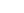. The position of the COM is uniformly probable everywhere, in agreement with the Heisenberg uncertainty principle.

Quantum mechanically the proper separation of the COM motion
is much more cumbersome than in classical mechanics. By introducing the coordinate vector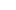of the center of mass as three of the degrees of freedom of the system and eliminating the coordinate vector of one (arbitrary) particle, so that the number of degrees of freedom stays the same, one obtains by a linear transformation a new set of coordinates ti. These coordinates are linear combinations of the old coordinates of all particles (nuclei and electrons). By applying the chain rule
Chain rule
In calculus, the chain rule is a formula for computing the derivative of the composition of two or more functions. That is, if f is a function and g is a function, then the chain rule expresses the derivative of the composite function in terms of the derivatives of f and g.In integration, the...

one can show that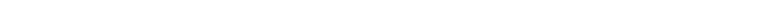The first term of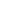is the kinetic energy of the COM motion, which can be treated separately since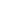does not depend on. As just stated, its eigenstates are plane waves. The constants 1/μij are positive and are linear combinations of all the inverse masses 1/mi. They are generalized reduced mass
Reduced mass
Reduced mass is the "effective" inertial mass appearing in the two-body problem of Newtonian mechanics. This is a quantity with the unit of mass, which allows the two-body problem to be solved as if it were a one-body problem. Note however that the mass determining the gravitational force is not...

es. The potential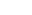consists of the Coulomb terms expressed in the new coordinates. The first term ofhas the usual appearance of a kinetic energy operator. The second term is known as the mass polarization term. The translationally invariant Hamiltoniancan be shown to be self-adjoint
In mathematics, an element x of a star-algebra is self-adjoint if x^*=x.A collection C of elements of a star-algebra is self-adjoint if it is closed under the involution operation...

and to be bounded from below. That is, its lowest eigenvalue is real and finite. Althoughis necessarily invariant under permutations of identical particles (sinceand the COM kinetic energy are invariant), its invariance is not manifest.

Not many actual molecular applications ofexist, see, however, the seminal work on the hydrogen molecule for an early application. In the great majority of computations of molecular wavefunctions the electronic
problem is solved with the clamped nucleus Hamiltonian arising in the first step of the Born-Oppenheimer approximation
Born-Oppenheimer approximation
In quantum chemistry, the computation of the energy and wavefunction of an average-size molecule is a formidable task that is alleviated by the Born–Oppenheimer approximation, named after Max Born and J. Robert Oppenheimer. For instance the benzene molecule consists of 12 nuclei and 42...

.

See Ref. for a thorough discussion of the mathematical properties of the Coulomb Hamiltonian. Also it is discussed in this paper whether one can arrive a priori at the concept of a molecule (as a stable system of electrons and nuclei with a well-defined geometry) from the properties of the Coulomb Hamiltonian alone.

## Clamped nucleus Hamiltonian

The clamped nucleus Hamiltonian describes the energy of the electrons in the electrostatic field of the nuclei, where the nuclei are assumed to be stationary with respect to an inertial frame.
The form of the electronic Hamiltonian is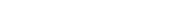The coordinates of electrons and nuclei are expressed with respect to a frame that moves
with the nuclei, so that the nuclei are at rest with respect to this frame. The frame stays parallel to a space-fixed frame. It is an inertial frame because the nuclei are assumed not to be accelerated by external forces or torques. The origin of the frame is arbitrary, it is usually positioned on a central nucleus or in the nuclear center of mass. Sometimes it is stated that the nuclei are "at rest in a space-fixed frame". This statement implies that the nuclei are viewed as classical particles, because a quantum mechanical particle cannot be at rest. (It would mean that it had simultaneously zero momentum and well-defined position, which contradicts Heisenberg's uncertainty principle).

Since the nuclear positions are constants, the electronic kinetic energy operator is invariant under translation over any nuclear vector. The Coulomb potential, depending on difference vectors, is invariant as well. In the description of atomic orbitals and the computation of integrals over atomic orbitals this invariance is used by equipping all atoms in the molecule with their own localized frames parallel to the space-fixed frame.

As explained in the article on the Born-Oppenheimer approximation
Born-Oppenheimer approximation
In quantum chemistry, the computation of the energy and wavefunction of an average-size molecule is a formidable task that is alleviated by the Born–Oppenheimer approximation, named after Max Born and J. Robert Oppenheimer. For instance the benzene molecule consists of 12 nuclei and 42...

, a sufficient number of solutions
of the Schrödinger equation of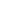leads to a potential energy surface
Potential energy surface
A potential energy surface is generally used within the adiabatic or Born–Oppenheimer approximation in quantum mechanics and statistical mechanics to model chemical reactions and interactions in simple chemical and physical systems...

(PES)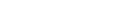. It is assumed that the functional dependence of V on its coordinates is such that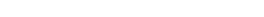for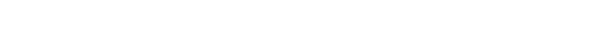where t and s are arbitrary vectors and Δφ is an infinitesimal angle,
Δφ >> Δφ2. This invariance condition on the PES is automatically fulfilled when the PES is expressed in terms of differences of, and angles between, the Ri, which is usually the case.

## Harmonic nuclear motion Hamiltonian

In the remaining part of this article we assume that the molecule is semi-rigid
Semi-rigid molecule
A semi-rigid molecule is a molecule which has a potential energy surface with a well-defined minimum corresponding to a stable structure of the molecule...

. In the second step of the BO approximation the nuclear kinetic energy Tn is reintroduced and the Schrödinger equation with Hamiltonian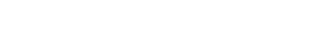is considered. One would like to recognize in its solution: the motion of the nuclear center of mass (3 degrees of freedom), the overall rotation of the molecule (3 degrees of freedom), and the nuclear vibrations. In general, this is not possible with the given nuclear kinetic energy, because it does not separate explicitly the 6 external degrees of freedom (overall translation and rotation) from the 3N-6 internal degrees of freedom. In fact, the kinetic energy operator here
is defined with respect to a space-fixed (SF) frame. If we were to move the origin of the SF frame to the nuclear center of mass, then, by application of the chain rule
Chain rule
In calculus, the chain rule is a formula for computing the derivative of the composition of two or more functions. That is, if f is a function and g is a function, then the chain rule expresses the derivative of the composite function in terms of the derivatives of f and g.In integration, the...

, nuclear mass polarization terms would appear. It is customary to ignore these terms altogether and we will follow this custom.

In order to achieve a separation we must distinguish internal and external coordinates, to which end Eckart introduced conditions
Eckart conditions
The Eckart conditions, named after Carl Eckart, sometimes referred to as Sayvetz conditions, simplify the nuclear motion Schrödinger equation that arises in the second step of the Born-Oppenheimer approximation. The Eckart conditions allow to a large extent the separation of the external ...

to be satisfied by the coordinates. We will
show how these conditions arise in a natural way from a harmonic analysis in mass-weighted Cartesian coordinates.

In order to simplify the expression for the kinetic energy we introduce mass-weighted displacement coordinates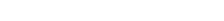.
Since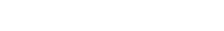the kinetic energy operator becomes,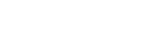If we make a Taylor expansion of V around the equilibrium geometry,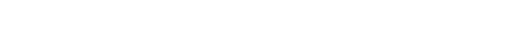and truncate after three terms (the so-called harmonic approximation), we can describe V with only the third term. The term V0 can be absorbed in the energy (gives a new zero of energy). The second term
is vanishing because of the equilibrium condition.
The remaining term contains the Hessian matrix
Hessian matrix
In mathematics, the Hessian matrix is the square matrix of second-order partial derivatives of a function; that is, it describes the local curvature of a function of many variables. The Hessian matrix was developed in the 19th century by the German mathematician Ludwig Otto Hesse and later named...

F of V, which is symmetric and may be diagonalized with an orthogonal 3N x 3N matrix with constant elements: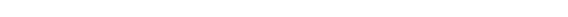It can be shown from the invariance of V under rotation and translation that six of the eigenvectors of F (last six rows of Q) have eigenvalue zero (are zero-frequency modes). They span the external space.
The first 3N-6 rows of Q are—for molecules in their ground state—eigenvectors with non-zero eigenvalue; they are the internal
coordinates and form an orthonormal basis for a 3N - 6 dimensional subspace of
the nuclear configuration space R3N, the internal space.
The zero-frequency eigenvectors are orthogonal to the eigenvectors of non-zero frequency.
It can be shown that these orthogonalities are in fact the Eckart conditions
Eckart conditions
The Eckart conditions, named after Carl Eckart, sometimes referred to as Sayvetz conditions, simplify the nuclear motion Schrödinger equation that arises in the second step of the Born-Oppenheimer approximation. The Eckart conditions allow to a large extent the separation of the external ...

. The kinetic
energy expressed in the internal coordinates is the internal (vibrational) kinetic energy.

With the introduction of normal coordinates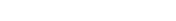the vibrational (internal) part of the Hamiltonian for the nuclear motion becomes in the harmonic approximation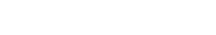The corresponding Schrödinger equation is easily solved, it factorizes into 3N-6 equations for one-dimensional harmonic oscillator
Harmonic oscillator
In classical mechanics, a harmonic oscillator is a system that, when displaced from its equilibrium position, experiences a restoring force, F, proportional to the displacement, x: \vec F = -k \vec x \, where k is a positive constant....

s. The main effort in this approximate solution of the nuclear motion Schrödinger equation is the computation of the Hessian F of V and its diagonalization.

This approximation to the nuclear motion problem, described in 3N mass-weighted Cartesian coordinates, became standard in quantum chemistry
Quantum chemistry
Quantum chemistry is a branch of chemistry whose primary focus is the application of quantum mechanics in physical models and experiments of chemical systems...

, since the days (1980s-1990s) that algorithms for accurate computations of the Hessian F became available. Apart from the harmonic approximation, it has as a further deficiency that the external (rotational and translational) motions of the molecule are not accounted for. They are accounted for in a rovibrational Hamiltonian
that sometimes is called Watson's Hamiltonian.

## Watson's nuclear motion Hamiltonian

In order to obtain a Hamiltonian for external (translation and rotation) motions coupled
to the internal (vibrational) motions, it is common to return at this point to classical mechanics and to formulate the classical kinetic energy corresponding to these motions of the nuclei. Classically it is easy to separate the translational—center of mass—motion from the other motions. However, the separation of the rotational from the vibrational motion is more difficult and is not completely possible. This ro-vibrational separation was first achieved by Eckart in 1935 by imposing by what is now known as Eckart conditions
Eckart conditions
The Eckart conditions, named after Carl Eckart, sometimes referred to as Sayvetz conditions, simplify the nuclear motion Schrödinger equation that arises in the second step of the Born-Oppenheimer approximation. The Eckart conditions allow to a large extent the separation of the external ...

. Since the problem is described in a frame (an "Eckart" frame) that rotates with the molecule, and hence is a non-inertial frame, energies associated with the fictitious force
Fictitious force
A fictitious force, also called a pseudo force, d'Alembert force or inertial force, is an apparent force that acts on all masses in a non-inertial frame of reference, such as a rotating reference frame....

s: centrifugal
Centrifugal force
Centrifugal force can generally be any force directed outward relative to some origin. More particularly, in classical mechanics, the centrifugal force is an outward force which arises when describing the motion of objects in a rotating reference frame...

and Coriolis force
Coriolis effect
In physics, the Coriolis effect is a deflection of moving objects when they are viewed in a rotating reference frame. In a reference frame with clockwise rotation, the deflection is to the left of the motion of the object; in one with counter-clockwise rotation, the deflection is to the right...

appear in the kinetic energy.

In general, the classical kinetic energy T defines the metric tensor g = (gij) associated with the curvilinear coordinates
Curvilinear coordinates
Curvilinear coordinates are a coordinate system for Euclidean space in which the coordinate lines may be curved. These coordinates may be derived from a set of Cartesian coordinates by using a transformation that is locally invertible at each point. This means that one can convert a point given...

s = (si) through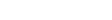.

The quantization step is the transformation of this classical kinetic energy into a quantum mechanical operator. It is common to follow Podolsky by writing down the Laplace-Beltrami operator
Laplace-Beltrami operator
In differential geometry, the Laplace operator, named after Pierre-Simon Laplace, can be generalized to operate on functions defined on surfaces in Euclidean space and, more generally, on Riemannian and pseudo-Riemannian manifolds. This more general operator goes by the name Laplace–Beltrami...

in the same (generalized, curvilinear) coordinates s as used for the classical form. The equation for this operator requires the inverse of the metric tensor g and its determinant. Multiplication of the Laplace-Beltrami operator bygives the required quantum mechanical kinetic energy operator. When we apply this recipe to Cartesian coordinates, which have unit metric, the same kinetic energy is obtained as by application of the quantization rules.

The nuclear motion Hamiltonian was obtained by Wilson and Howard in 1936,
who followed this procedure, and further refined by Darling and Dennison in 1940. It remained the standard until 1968, when Watson was able to simplify it drastically by commuting through the derivatives the determinant of the metric tensor. We will give the ro-vibrational Hamiltonian obtained by Watson,
which often is referred to as the Watson Hamiltonian. Before we do this we must mention
that a derivation of this Hamiltonian is also possible by starting from the Laplace operator
in Cartesian form, application of coordinate transformations, and use of the chain rule.
The Watson Hamiltonian, describing all motions of the N nuclei, is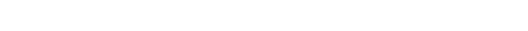The first term is the center of mass term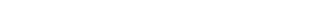The second term is the rotational term akin to the kinetic energy of the rigid rotor
Rigid rotor
The rigid rotor is a mechanical model that is used to explain rotating systems.An arbitrary rigid rotor is a 3-dimensional rigid object, such as a top. To orient such an object in space three angles are required. A special rigid rotor is the linear rotor which isa 2-dimensional object, requiring...

. Here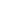is the α component of the body-fixed rigid rotor angular momentum operator,
Euler angles
The Euler angles are three angles introduced by Leonhard Euler to describe the orientation of a rigid body. To describe such an orientation in 3-dimensional Euclidean space three parameters are required...

. The operator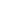is a component of an operator known
as the vibrational angular momentum operator (although it does not satisfy angular momentum commutation relations),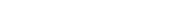with the Coriolis coupling constant: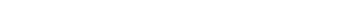Here εαβγ is the Levi-Civita symbol
Levi-Civita symbol
The Levi-Civita symbol, also called the permutation symbol, antisymmetric symbol, or alternating symbol, is a mathematical symbol used in particular in tensor calculus...

. The terms quadratic in theare centrifugal terms, those bilinear inand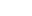are Coriolis terms.
The quantities Q s, iγ are the components of the normal coordinates introduced above.
Alternatively, normal coordinates may be obtained by application of Wilson's GF method
GF method
The GF method, sometimes referred to as FG method, is a classical mechanical method introduced by E. Bright Wilson to obtain certain internal coordinates fora vibrating semi-rigid molecule, the so-called normal coordinates Qk...

.
The 3 x 3 symmetric matrix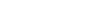is called the effective reciprocal inertia tensor. If all q s were zero (rigid molecule) the Eckart frame would coincide with a principal axes frame (see rigid rotor
Rigid rotor
The rigid rotor is a mechanical model that is used to explain rotating systems.An arbitrary rigid rotor is a 3-dimensional rigid object, such as a top. To orient such an object in space three angles are required. A special rigid rotor is the linear rotor which isa 2-dimensional object, requiring...

) andwould be diagonal, with the equilibrium reciprocal moments of inertia on the diagonal. If all q s would be zero, only the kinetic energies of translation and rigid rotation would survive.

The potential-like term U is the Watson term: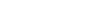proportional to the trace of the effective reciprocal inertia tensor.

The fourth term in the Watson Hamiltonian is the kinetic
energy associated with the vibrations of the atoms (nuclei) expressed in normal coordinates qs, which as stated above, are given in terms of nuclear displacements ρ by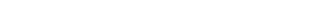Finally V is the unexpanded potential energy by definition depending on internal coordinates only. In the harmonic approximation it takes the form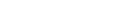• Quantum chemistry computer programs
Quantum chemistry computer programs
Quantum chemistry computer programs are used in computational chemistry to implement the methods of quantum chemistry. Most include the Hartree–Fock and some post-Hartree–Fock methods. They may also include density functional theory , molecular mechanics or semi-empirical quantum...

• Franck-Condon principle
Franck-Condon principle
The Franck–Condon principle is a rule in spectroscopy and quantum chemistry that explains the intensity of vibronic transitions. Vibronic transitions are the simultaneous changes in electronic and vibrational energy levels of a molecule due to the absorption or emission of a photon of the...

• Born-Oppenheimer approximation
Born-Oppenheimer approximation
In quantum chemistry, the computation of the energy and wavefunction of an average-size molecule is a formidable task that is alleviated by the Born–Oppenheimer approximation, named after Max Born and J. Robert Oppenheimer. For instance the benzene molecule consists of 12 nuclei and 42...

• GF method
GF method
The GF method, sometimes referred to as FG method, is a classical mechanical method introduced by E. Bright Wilson to obtain certain internal coordinates fora vibrating semi-rigid molecule, the so-called normal coordinates Qk...

• Eckart conditions
Eckart conditions
The Eckart conditions, named after Carl Eckart, sometimes referred to as Sayvetz conditions, simplify the nuclear motion Schrödinger equation that arises in the second step of the Born-Oppenheimer approximation. The Eckart conditions allow to a large extent the separation of the external ...

• Rigid rotor
Rigid rotor
The rigid rotor is a mechanical model that is used to explain rotating systems.An arbitrary rigid rotor is a 3-dimensional rigid object, such as a top. To orient such an object in space three angles are required. A special rigid rotor is the linear rotor which isa 2-dimensional object, requiring...